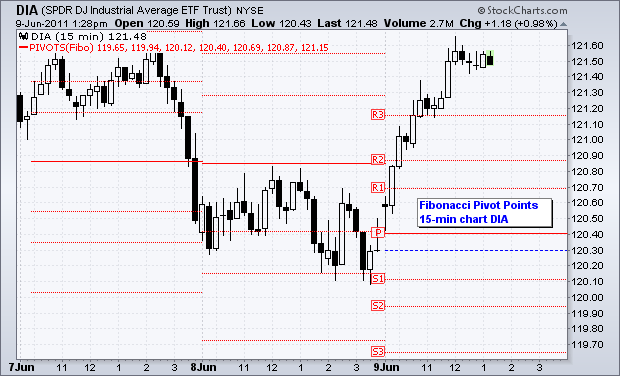# Fibonacci Pivot Points

Summary: In this article we describe the use and calculation of Fibonacci Pivot Points, a little known variant compared with traditional pivot points. The novelty about this tool is that it interestingly combines the pivots and Fibonacci sequence to determine support and resistance levels where there is high probability that the market made ​​major moves.

The pivot points in its different variations can be used in two ways: to display the main trend in the market that we are analyzing or to display price levels in which we can open and close a position. To identify the direction, if the price level of the pivot point is crossed in an upward motion, then we can say that the market is bullish, but if the break occurs in a downward movement, then the market is bearish. Generally, if prices remain above the pivot point then the upward trend will continue until an event cause a reverse in the price movement and when the price remains below this level then the market will continue its downward direction until something happens that force a change in the current trend.

At this point we must bear in mind that the pivot points are indicators mainly used for short-term trends analysis, and can be useful during the day in which are calculated and therefore need to be redefined at the end of each trading day.

One of the biggest advantages of pivote points is that they can provide entry and exit points easy to visualize and can be included in any trading strategy. For example, we can place a limit order at a support level to be executed if this level is crossed or we can use a stop loss in one of the resistances.

A popular but less common variant of pivot points is based on Fibonacci formulas. In Fibonacci pivot points, the main pivot is calculated by adding the maximum (or higher), minimum (or low) and end of the period we wish to analyze, and then we divide the result by 3. The range is the difference between the maximum and minimum (high – low), and successive levels are projected by adding a sequence of Fibonacci to the pivot, and multiplying the result by the range.

## Fibonacci pivot points formulas### Standard model

• R3 = Pivot + 1.000 x (High – Low)
• R2 = Pivot + 0.618 x (High – Low)
• R1 = Pivot + 0.382 x (High – Low)
• Pivot = (High + Low + Close) / 3
• S1 = Pivot – 0.382 x (High – Low)
• S2 = Pivot – 0.618 x (High – Low)
• S3 = Pivot 1000 x (High – Low)

There is also a different version of this formula that uses the value 50% for the second levels of support and resistance and also uses the closing price for computations instead of the pivot. The range of the previous period is also calculated and multiplied by the three successive Fibonacci levels, and the result is added to the closing price for the resistances or subtracted from the closing price for the support levels.

The process of calculating this Fibonacci pivot points variant is shown below:

Step 1:

• Range 1 extension = 1,382 x Range
• Range 2 extension = 1.5 x Range
• Range 3 extension = 1,618 x Range

Step 2:

• Fibonacci resistance level 1 = Range 1 extension + Closing prince of the previous period.
• Fibonacci resistance Level 2 =Range 2 extension + Closing prince of the previous period.
• Fibonacci resistance level 3 = Range 3 extension + Closing prince of the previous period.

Step 3:

• Fibonacci support level 1 = Closing price of the previous period – Range 1 extension
• Fibonacci support level 2 = Closing price of the previous period – Range 2 extension
• Fibonacci support level 3 = Closing price of the previous period – Range 3 extension

The Fibonacci pivot points can be used as traditional pivot points to establish levels where it is likely that strong price movements due to bounces or breaks of support and resistance will occur.